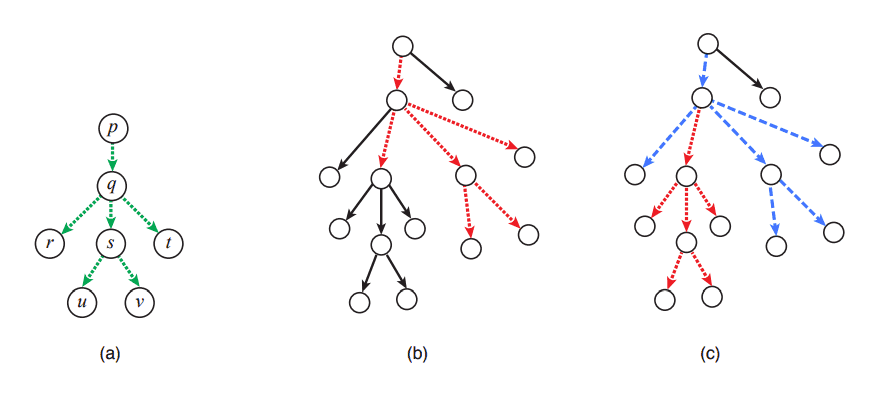### I - Ink Colors

##### Languages: MS C# .NET 4.7.2053,GNU G++11 5.1.0 ...

Stick Man left the family tree and went out for adventures. On his journey he found a strange tree with the root on the air and branches directed towards the ground. He decided to paint some of the tree branches to remind himself of home. To do so he wants that branches painted with the same color are all connected and form a stick man. A stick man is a group of six branches $(p,q)$ $(q,r)$ $(q,s)$ $(q,t)$ $(s,u)$ and $(s,v)$, as show in figure (a) below. Figure (b) shows a tree with one stick man painted and figure (c) shows the same tree with two stick men painted.Stick Man would like to paint as many stick men on the tree as possible, such that each branch is part of at most a single stick man. Can you help him figure out how many ink colors he needs to buy?

#### Input

The first line contains an integer $N$ ($1 \leq N \leq 10^5$) indicating the number of nodes in the tree. Nodes are identified by distinct integers from $1$ to $N$, where node $1$ is the root of the tree. The second line contains $N-1$ integers $P_2, P_3, ..., P_N$ ($1 \leq P_i \leq N$ for $i = 2, 3, ..., N$), where the value $P_i$ represents that there is a branch $(P_i,i)$, that is, from node $P_i$ to node $i$.

#### Output

Output a single line with an integer indicating the maximum number of stick men that might be simultaneously painted on the tree.

#### Sample test(s)

Input
14 1 1 2 2 2 2 5 5 5 6 6 9 9
Output
2
Input
13 13 7 5 1 5 2 5 7 4 2 2 4
Output
2